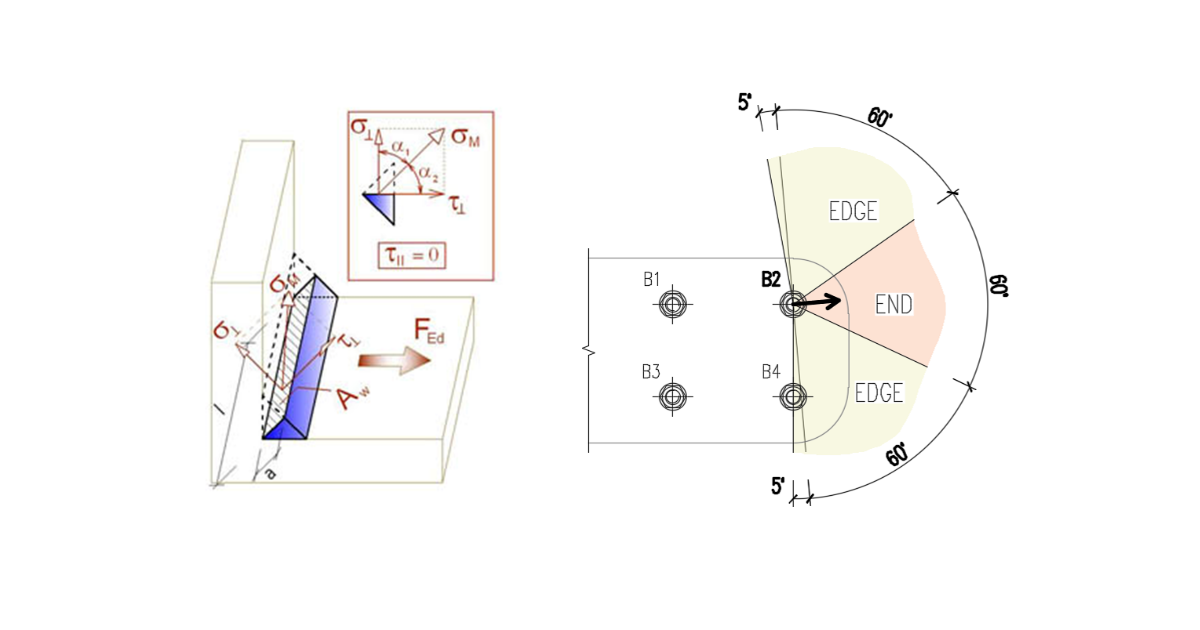### Choose language# Detailing of bolts and welds according to Hong Kong Code

$$BoltsBolt minimum spacing is according to Cl. 9.3.1.1: Centre to centre of bolt should be larger than $$2.5 \cdot d$$, where $$d$$ is nominal bolt diameter.Minimum edge distance measured from the centreline of the bolt is according to Table 9.3: Bolt sizeMinimum edge distance [mm] M1218 M1622 M1824 M2026 M2228 M24 and over$$1.25 \cdot d$$ WeldsMinimum leg size of fillet welds is checked according to Table 9.1. Thickness of the thickest part [mm]Minimum leg length [mm]Minimum throat thickness [mm] $$t \le 6$$32.121 $$6 < t \le 13$$53.536 $$13 < t \le 19$$64.243 $$19 > t$$85.657$$